## Example Questions

### Example Question #1 : How To Solve For A Variable As Part Of A Fraction

Solve for.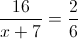Possible Answers: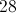Correct answer:Explanation:

Cross multiply.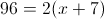Dsitribute.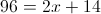Solve for.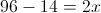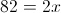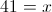### Example Question #2 : How To Solve For A Variable As Part Of A Fraction

The quotient of a fraction is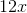. If the numerator is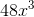, what is the value of the denominator?

Possible Answers: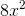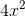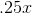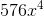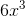Correct answer:Explanation:

Step 1: Set up the equation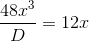Step 2: Solve for D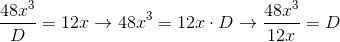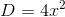### Example Question #3 : How To Solve For A Variable As Part Of A Fraction

Solve for: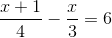Possible Answers: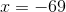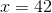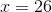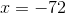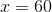Correct answer:Explanation:

Solve for x:Step 1: Find the least common denominator,, and adjust the fractions accordingly: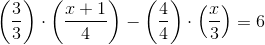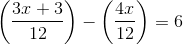Solve for: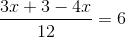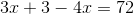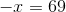### Example Question #1 : How To Solve For A Variable As Part Of A Fraction

If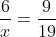, then what is the value of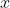?

Possible Answers:

38/3

none of these

9/114

7/12

3/38

Correct answer:

38/3

Explanation:

cross multiply:

(6)(19) = 9x

114=9x

x = 38/3

### Example Question #1 : How To Solve For A Variable As Part Of A Fraction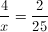Find x.

Possible Answers: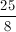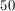None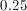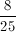Correct answer:Explanation:

Cross multiply: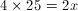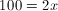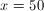### Example Question #2 : How To Solve For A Variable As Part Of A Fraction

The numerator of a fraction is the sum of 4 and 5 times the denominator. If you divide the fraction by 2, the numerator is 3 times the denominator. Find the simplified version of the fraction.

Possible Answers:Correct answer:Explanation:

Let numerator = N and denominator = D.

According to the first statement,

N = (D x 5) + 4.

According to the second statement, N / 2 = 3 * D.

Let's multiply the second equation by –2 and add itthe first equation:

–N = –6D

+[N = (D x 5) + 4]

=

–6D + (D x 5) + 4 = 0

–1D + 4 = 0

D = 4

Thus, N = 24.

Therefore, N/D = 24/4 = 6.

### Example Question #7 : How To Solve For A Variable As Part Of A Fraction

Solve the following equation for the given variable: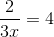Possible Answers: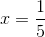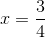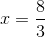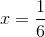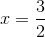Correct answer:Explanation:

To solve this equation we have to multiply both sides by the denominator to get rid of the fraction.

Doing this yields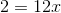Then to solve the last step is to isolate the variable by dividing both sides by 12.
Thus,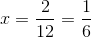.

### Example Question #8 : How To Solve For A Variable As Part Of A Fraction

For what value ofis the equation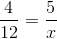true?

Possible Answers:Correct answer:Explanation:

When the equation is cross multiplied, it becomes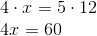.

Hence,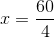, or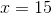### Example Question #9 : How To Solve For A Variable As Part Of A Fraction

Solve the following equation for: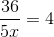.

Reduce any fractions in your final answer.

Possible Answers: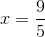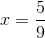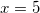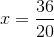Correct answer:Explanation:

To solve an equation with a variable in a fraciton, treat the denominator as a constant value and multiply both sides of the equation by the denominator in order to eliminate it.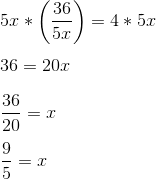### Example Question #10 : How To Solve For A Variable As Part Of A Fraction

Solve for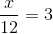Possible Answers: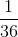Correct answer:Explanation:

To find the answer, multiply the right side by. The result is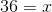.

### All ACT Math Resources